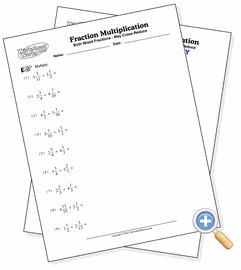# With Two Mixed Fractions

## Fraction MultiplicationDevelop skills multiplying two mixed fractions

These problems involve the multiplication of two mixed fractions. This provides the most difficult fraction multiplication problem since it involves converting to improper fractions, cross-reducing, multiplying and finally simplifying in each problem.